# Absorption Resistance Notes | Study Mass Transfer - Chemical Engineering

## Chemical Engineering: Absorption Resistance Notes | Study Mass Transfer - Chemical Engineering

The document Absorption Resistance Notes | Study Mass Transfer - Chemical Engineering is a part of the Chemical Engineering Course Mass Transfer.
All you need of Chemical Engineering at this link: Chemical Engineering

4.9.5. Absorption resistance
During absorption, when transport from the bulk of the gas phase to that of the liquid phase, the possibility of the transport resistance arising on the gas side. This is relatively infrequent occurrence in absorption process. But it can be found under certain circumstances where fast and instantaneous reactions are involved, especially when combined with low gas phase of the reactant.
The absorption rate for fast pseudo-fast-order reaction is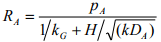(4.76)
The relation for instantaneous reaction is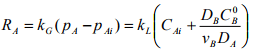(4.77)
Using Henry’s law (pAi=HcAi), the interface concentration can be removed from Equation (4.77) to give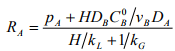(4.78)
when the reaction is at the interface: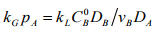(4.79)
If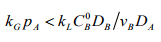(4.80)
then transport resistance is mainly on the gas side and the absorption rate is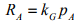(4.81)

Example Problem 4.4:
In a reactor Carbon dioxide is absorbed in NaOH. The CO2 partial pressure ranges from 2.5 bar at the reaction inlet and (0.025) at the outlet. The liquid phase has a roughly constant composition of 0.96 M NaOH. Find out the absorption rate at reactor inlet.
Data: kL = 0.025 cm/s, kG = 2.5×10-5 mol.cm-2s-1bar-1 , H = 56.2 bar l mol-1 , DCO2 = 1.6×10-5 cm2s-1 , DOH/DCO2 = 1.7, k2C0OH = 7000S-1 , C0OH = 0.40

Solution 4.4:
Parameter M is calculated as an initial step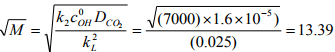The enhancement factor Ei is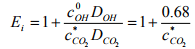As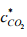= p/H, it follows that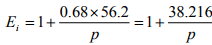Hence, Ei = 16.28 at the reactor inlet and Ei = 1528.64 at the outlet. E is determined iteratively from the Wellek’s equation In the present problem its value is 3.492. Hence, absorption rate at the reactor inlet is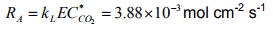Nomenclature
ā  Specific interfacial contact area between gas and liquid, m/m3
Ls  Liquid flow rate per unit area basis, (solute free basis) mol/h.m2
Ā, An  Absorption factor M Parameter defined in Equation (4.77)
G/  Gas flow rate per unit area basis, mol/h.m
NtG   Number of gas phase transfer units
Gs   Gas flow rate per unit area basis,(solute free basis) mol/h.m2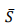Stripping factor
hT Packing height, m
U Overall gas phase conversion
HtG Height if transfer units, m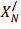Moles of Nth component in the liquid stream per mole of solvent entering the absorber
KY Overall gas phase mass transfer coefficient, kmol/m2h (ΔX)
x, y Mole fraction of solute in liquid and gas
kx, ky Individual gas phase mass transfer coefficients, kmol/m2 h (ΔX)
X, Y Mole ratio of solute in liquid and gas
KG Overall gas phase mass transfer coefficient, kmol/m2 h (Δp)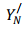Moles of Nth component in the gas stream leaving any plate
L/ Liquid flow rate per unit area basis, mol/h.m2

The document Absorption Resistance Notes | Study Mass Transfer - Chemical Engineering is a part of the Chemical Engineering Course Mass Transfer.
All you need of Chemical Engineering at this link: Chemical EngineeringUse Code STAYHOME200 and get INR 200 additional OFF

## Mass Transfer

29 videos|45 docs|44 tests

Track your progress, build streaks, highlight & save important lessons and more!

,

,

,

,

,

,

,

,

,

,

,

,

,

,

,

,

,

,

,

,

,

;